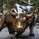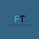37821 views
//Author - Rajandran R
//www.marketcalls.in
study("Supertrend V1.0 - Buy or Sell Signal", overlay = true)

Factor=input(3, minval=1,maxval = 100)
Pd=input(7, minval=1,maxval = 100)

Up=hl2-(Factor*atr( Pd ))
Dn=hl2+(Factor*atr( Pd ))

TrendUp=close>TrendUp? max(Up,TrendUp) : Up
TrendDown=close<TrendDown? min(Dn,TrendDown) : Dn

Trend = close > TrendDown ? 1: close< TrendUp? -1: nz (Trend,1)
Tsl = Trend==1? TrendUp: TrendDown

linecolor = Trend == 1 ? green : red

plot( Tsl , color = linecolor , style = line , linewidth = 2,title = "SuperTrend")

plotshape(cross(close, Tsl ) and close>Tsl , "Up Arrow", shape.triangleup,location.belowbar,green,0,0)
plotshape(cross( Tsl ,close) and close<Tsl , "Down Arrow", shape.triangledown , location.abovebar, red,0,0)
//plot(Trend==1 and Trend==-1,color = linecolor, style = circles, linewidth = 3,title="Trend")

plotarrow(Trend == 1 and Trend == -1 ? Trend : na, title="Up Entry Arrow", colorup=lime, maxheight=60, minheight=50, transp=0)
plotarrow(Trend == -1 and Trend == 1 ? Trend : na, title="Down Entry Arrow", colordown=red, maxheight=60, minheight=50, transp=0)

## KommentareHello, I can't use alert on this indicator. I tried but doesn not work.

Anybody could help me?

Thank you!
AntwortenHi There the alert chart is a little confusing, did anyone evr figure outhow to set the alerts for up and down arrows? thanks
Antworten